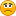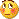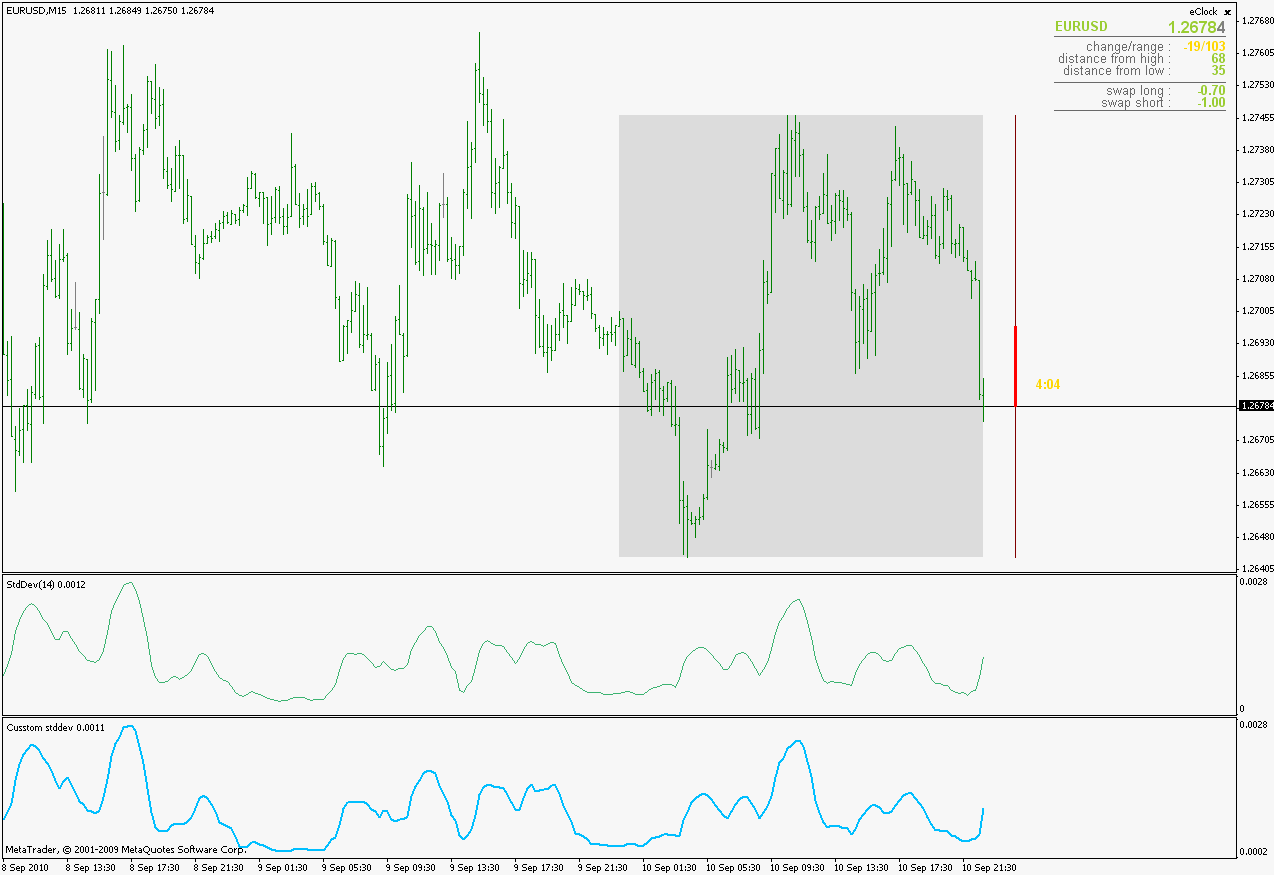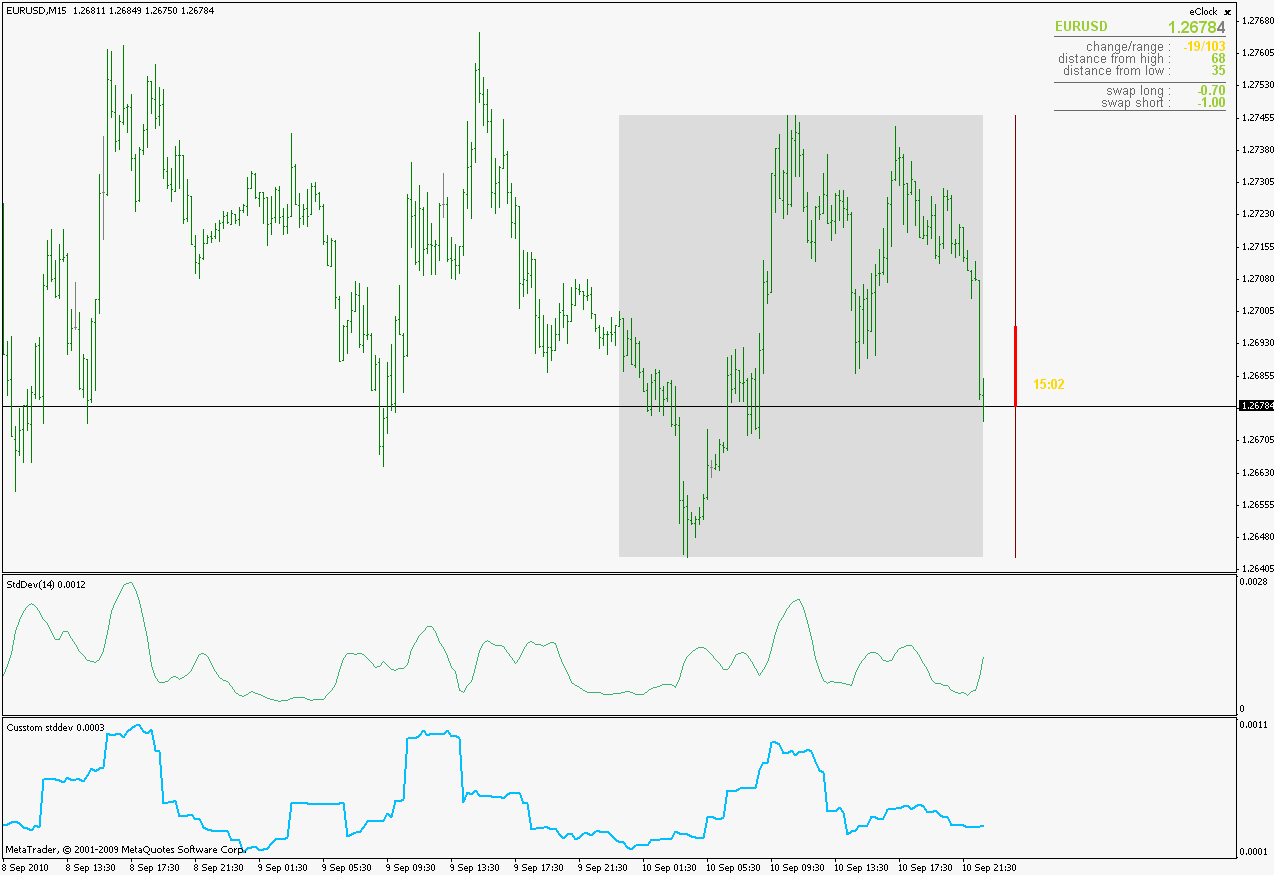Std Deviation - page 3151902

Unfortunately metatrader does not work like tradestation, and for some reason metatrader people enabled that feature from the built in indicators and did not do the same thing for custom indicatorsmzeki:

I tried what you said (really tried hard) but again failedCan you please post here the full code with changes.

Instead of mentioning any indicator (like RSI) inside the code, isn't it possible to use it for any indicator that the new indicator is attached to, just like Moving Average of Oscillator..151902

Little things that brings the joy back again:):)

mrtools:
Thank you Mladen,here's a picture of AllMa ZeroLag using kurtosis, verry,verry interesting!!!!!249

Not sure if this has been done before or if anyone will have any use for this but I put together a simple extension of the Std-Dev indicator to allow you to calculate a simple MA of the Std-Dev. I use this to gauge when price is trending, if SD is greater than the moving average then price is in a trend and you should enter an order in the direction of the movement. seems to work best with high Std values, I currently use 50 period Std and 15 moving average period. It works well as a filter for my current EA, it managed to increase the accuracy by about 15%. Hope this helps someone.

Files:6

Can spread and slippage be roughly estimated through standard deviation?

So slippage and spread increase in times of increased price fluctuation and news events. Can it be roughly estimated with regards to the standard deviation of past days or hours? For example. (S+S)=k*stdev(d1,d2,....dn) with k as a constant.1163

stdev formula help

Hi

I am have been trying to find out how to work out the Standard Deviation between the high and open of the previous bar.

Does anyone have some code to work this out.

any help would be great.

Cheers

Beno1152

Beno:
Hi

I am have been trying to find out how to work out the Standard Deviation between the high and open of the previous bar.

Does anyone have some code to work this out.

any help would be great.

Cheers

Beno

Hi beno,

not sure what you are exactly asking here. In simple terms Standard Deviation is a way of saying how far typical values are from mean. In your case you just have High and Open of previous bar you can average these values cause otherwise doesn't seem to make sense. If you are referring to STDEV of MT4 that is something else and is based on MA

-guyver1163

Hi guyver

This is all part of a system I have a developed in excel.

In excel if you have a high of 2.5968 and a low of 2.5706 the average is 2.5837

(2.5968+2.5706)/2=2.5837

But the stdev is 0.0185 I have been trying to create e script to emulat the same formula.

I hope this makes sense151902

Beno

To use high-low : use metatrader standard deviation indicator and in "Apply to" field use median price. Also use simple moving average in the "method" field (mean corresponds to simple moving average)

To use high-open : use the attached indicator. It calculates standard deviation of the (high + open)/2. Difference is not big compared to median price deviations (here is a comparison - lower is the custom one)___________________________

If it is not what you had in mind the simply change line 65 from this :

for(i=limit; i >= 0; i--) prices = (High+Open)/2.0;[/php]to any expression you would like deviations to be calculated for (price shift is used to adjust the shift you want, in your case from your first post it would be 1 and then it will calculate standard deviation of (high + open)/2 of previous bar) if I understood correctly the idea

___________________________

If you want a standard deviation of a difference of high and open, replace that line with this one :

[php]for(i=limit; i >= 0; i--) prices = (High-Open);
and in that case you are going to get something like thisFiles:1152

Beno:
Hi guyver

This is all part of a system I have a developed in excel.

In excel if you have a high of 2.5968 and a low of 2.5706 the average is 2.5837

(2.5968+2.5706)/2=2.5837

But the stdev is 0.0185 I have been trying to create e script to emulat the same formula.

I hope this makes sense

I used the word average because with less data there is really no meaningful information we can use through STDEV . So mean or average is fine for the scenario i wrote before imho but in your case

STD DEV..

To get std-dev we need to first get the variance which is another way of saying how the "data" is spread in other words the average of the distance of values from mean squared.. in your example using the same values we can write ( in a basic form ).

// mql4 code

double high = 2.5968;

double low = 2.5706;

double mean = (high+low)/2;

double variance = ((high-low)*(high-low))/2;

double stdev = MathSqrt(variance);

Comment(stdev);

//

As you can see first we take the average or mean cause we need the middle value then we take the variance which is says how the data is spread across mean and then we take the stddev which says how "far"are the typical values from the mean.

-- hope this helps

guyver28